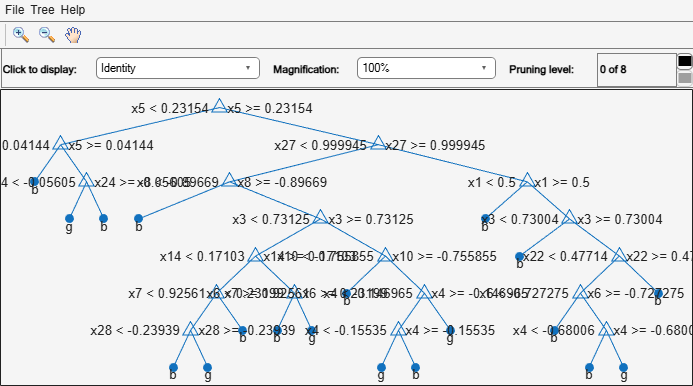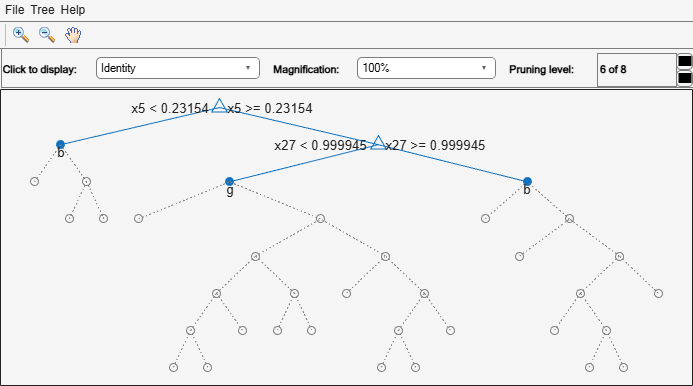## Improving Classification Trees and Regression Trees

You can tune trees by setting name-value pairs in `fitctree` and `fitrtree`. The remainder of this section describes how to determine the quality of a tree, how to decide which name-value pairs to set, and how to control the size of a tree.

### Examining Resubstitution Error

Resubstitution error is the difference between the response training data and the predictions the tree makes of the response based on the input training data. If the resubstitution error is high, you cannot expect the predictions of the tree to be good. However, having low resubstitution error does not guarantee good predictions for new data. Resubstitution error is often an overly optimistic estimate of the predictive error on new data.

#### Classification Tree Resubstitution Error

This example shows how to examine the resubstitution error of a classification tree.

`load fisheriris`

Train a default classification tree using the entire data set.

`Mdl = fitctree(meas,species);`

Examine the resubstitution error.

`resuberror = resubLoss(Mdl)`
```resuberror = 0.0200 ```

The tree classifies nearly all the Fisher iris data correctly.

### Cross Validation

To get a better sense of the predictive accuracy of your tree for new data, cross validate the tree. By default, cross validation splits the training data into 10 parts at random. It trains 10 new trees, each one on nine parts of the data. It then examines the predictive accuracy of each new tree on the data not included in training that tree. This method gives a good estimate of the predictive accuracy of the resulting tree, since it tests the new trees on new data.

#### Cross Validate a Regression Tree

This example shows how to examine the resubstitution and cross-validation accuracy of a regression tree for predicting mileage based on the `carsmall` data.

Load the `carsmall` data set. Consider acceleration, displacement, horsepower, and weight as predictors of MPG.

```load carsmall X = [Acceleration Displacement Horsepower Weight];```

Grow a regression tree using all of the observations.

`rtree = fitrtree(X,MPG);`

Compute the in-sample error.

`resuberror = resubLoss(rtree)`
```resuberror = 4.7188 ```

The resubstitution loss for a regression tree is the mean-squared error. The resulting value indicates that a typical predictive error for the tree is about the square root of 4.7, or a bit over 2.

Estimate the cross-validation MSE.

```rng 'default'; cvrtree = crossval(rtree); cvloss = kfoldLoss(cvrtree)```
```cvloss = 25.6450 ```

The cross-validated loss is almost 25, meaning a typical predictive error for the tree on new data is about 5. This demonstrates that cross-validated loss is usually higher than simple resubstitution loss.

### Choose Split Predictor Selection Technique

The standard CART algorithm tends to select continuous predictors that have many levels. Sometimes, such a selection can be spurious and can also mask more important predictors that have fewer levels, such as categorical predictors. That is, the predictor-selection process at each node is biased. Also, standard CART tends to miss the important interactions between pairs of predictors and the response.

To mitigate selection bias and increase detection of important interactions, you can specify usage of the curvature or interaction tests using the `'PredictorSelection'` name-value pair argument. Using the curvature or interaction test has the added advantage of producing better predictor importance estimates than standard CART.

This table summarizes the supported predictor-selection techniques.

Technique`'PredictorSelection'` ValueDescriptionTraining speedWhen to specify
Standard CART Default

Selects the split predictor that maximizes the split-criterion gain over all possible splits of all predictors.

Baseline for comparison

Specify if any of these conditions are true:

• All predictors are continuous

• Predictor importance is not the analysis goal

• For boosting decision trees

Curvature test `'curvature'`Selects the split predictor that minimizes the p-value of chi-square tests of independence between each predictor and the response.Comparable to standard CART

Specify if any of these conditions are true:

• The predictor variables are heterogeneous

• Predictor importance is an analysis goal

• Enhance tree interpretation

Interaction test `'interaction-curvature'`Chooses the split predictor that minimizes the p-value of chi-square tests of independence between each predictor and the response (that is, conducts curvature tests), and that minimizes the p-value of a chi-square test of independence between each pair of predictors and response.Slower than standard CART, particularly when data set contains many predictor variables.

Specify if any of these conditions are true:

• The predictor variables are heterogeneous

• You suspect associations between pairs of predictors and the response

• Predictor importance is an analysis goal

• Enhance tree interpretation

For more details on predictor selection techniques:

### Control Depth or “Leafiness”

When you grow a decision tree, consider its simplicity and predictive power. A deep tree with many leaves is usually highly accurate on the training data. However, the tree is not guaranteed to show a comparable accuracy on an independent test set. A leafy tree tends to overtrain (or overfit), and its test accuracy is often far less than its training (resubstitution) accuracy. In contrast, a shallow tree does not attain high training accuracy. But a shallow tree can be more robust — its training accuracy could be close to that of a representative test set. Also, a shallow tree is easy to interpret. If you do not have enough data for training and test, estimate tree accuracy by cross validation.

`fitctree` and `fitrtree` have three name-value pair arguments that control the depth of resulting decision trees:

• `MaxNumSplits` — The maximal number of branch node splits is `MaxNumSplits` per tree. Set a large value for `MaxNumSplits` to get a deep tree. The default is `size(X,1) – 1`.

• `MinLeafSize` — Each leaf has at least `MinLeafSize` observations. Set small values of `MinLeafSize` to get deep trees. The default is `1`.

• `MinParentSize` — Each branch node in the tree has at least `MinParentSize` observations. Set small values of `MinParentSize` to get deep trees. The default is `10`.

If you specify `MinParentSize` and `MinLeafSize`, the learner uses the setting that yields trees with larger leaves (i.e., shallower trees):

```MinParent = max(MinParentSize,2*MinLeafSize)```

If you supply `MaxNumSplits`, the software splits a tree until one of the three splitting criteria is satisfied.

For an alternative method of controlling the tree depth, see Pruning.

#### Select Appropriate Tree Depth

This example shows how to control the depth of a decision tree, and how to choose an appropriate depth.

Load the `ionosphere` data.

`load ionosphere`

Generate an exponentially spaced set of values from `10` through `100` that represent the minimum number of observations per leaf node.

`leafs = logspace(1,2,10);`

Create cross-validated classification trees for the `ionosphere` data. Specify to grow each tree using a minimum leaf size in `leafs`.

```rng('default') N = numel(leafs); err = zeros(N,1); for n=1:N t = fitctree(X,Y,'CrossVal','On',... 'MinLeafSize',leafs(n)); err(n) = kfoldLoss(t); end plot(leafs,err); xlabel('Min Leaf Size'); ylabel('cross-validated error');```The best leaf size is between about `20` and `50` observations per leaf.

Compare the near-optimal tree with at least `40` observations per leaf with the default tree, which uses `10` observations per parent node and `1` observation per leaf.

```DefaultTree = fitctree(X,Y); view(DefaultTree,'Mode','Graph')``````OptimalTree = fitctree(X,Y,'MinLeafSize',40); view(OptimalTree,'mode','graph')``````resubOpt = resubLoss(OptimalTree); lossOpt = kfoldLoss(crossval(OptimalTree)); resubDefault = resubLoss(DefaultTree); lossDefault = kfoldLoss(crossval(DefaultTree)); resubOpt,resubDefault,lossOpt,lossDefault```
```resubOpt = 0.0883 ```
```resubDefault = 0.0114 ```
```lossOpt = 0.1054 ```
```lossDefault = 0.1111 ```

The near-optimal tree is much smaller and gives a much higher resubstitution error. Yet, it gives similar accuracy for cross-validated data.

### Pruning

Pruning optimizes tree depth (leafiness) by merging leaves on the same tree branch. Control Depth or “Leafiness” describes one method for selecting the optimal depth for a tree. Unlike in that section, you do not need to grow a new tree for every node size. Instead, grow a deep tree, and prune it to the level you choose.

Prune a tree at the command line using the `prune` method (classification) or `prune` method (regression). Alternatively, prune a tree interactively with the tree viewer:

`view(tree,'mode','graph')`

To prune a tree, the tree must contain a pruning sequence. By default, both `fitctree` and `fitrtree` calculate a pruning sequence for a tree during construction. If you construct a tree with the `'Prune'` name-value pair set to `'off'`, or if you prune a tree to a smaller level, the tree does not contain the full pruning sequence. Generate the full pruning sequence with the `prune` method (classification) or `prune` method (regression).

#### Prune a Classification Tree

This example creates a classification tree for the `ionosphere` data, and prunes it to a good level.

Load the `ionosphere` data:

`load ionosphere`

Construct a default classification tree for the data:

`tree = fitctree(X,Y);`

View the tree in the interactive viewer:

`view(tree,'Mode','Graph')`Find the optimal pruning level by minimizing cross-validated loss:

```[~,~,~,bestlevel] = cvLoss(tree,... 'SubTrees','All','TreeSize','min')```
```bestlevel = 6 ```

Prune the tree to level `6`:

`view(tree,'Mode','Graph','Prune',6)`Alternatively, use the interactive window to prune the tree.

The pruned tree is the same as the near-optimal tree in the "Select Appropriate Tree Depth" example.

Set `'TreeSize'` to `'SE'` (default) to find the maximal pruning level for which the tree error does not exceed the error from the best level plus one standard deviation:

`[~,~,~,bestlevel] = cvLoss(tree,'SubTrees','All')`
```bestlevel = 6 ```

In this case the level is the same for either setting of `'TreeSize'`.

Prune the tree to use it for other purposes:

```tree = prune(tree,'Level',6); view(tree,'Mode','Graph')```Breiman, L., J. H. Friedman, R. A. Olshen, and C. J. Stone. Classification and Regression Trees. Boca Raton, FL: Chapman & Hall, 1984.

 Loh, W.Y. and Y.S. Shih. “Split Selection Methods for Classification Trees.” Statistica Sinica, Vol. 7, 1997, pp. 815–840.

 Loh, W.Y. “Regression Trees with Unbiased Variable Selection and Interaction Detection.” Statistica Sinica, Vol. 12, 2002, pp. 361–386.